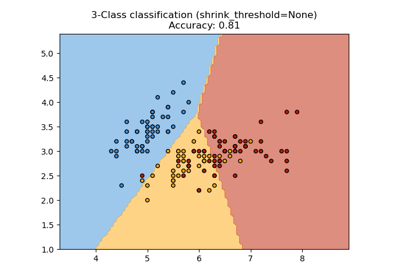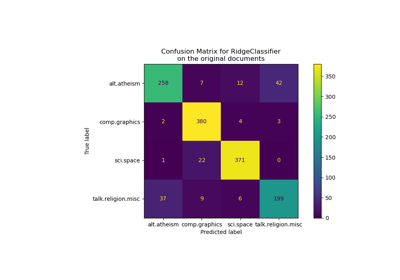# sklearn.neighbors.NearestCentroid¶

class sklearn.neighbors.NearestCentroid(metric='euclidean', *, shrink_threshold=None)[source]

Nearest centroid classifier.

Each class is represented by its centroid, with test samples classified to the class with the nearest centroid.

Read more in the User Guide.

Parameters:
metricstr or callable, default=”euclidean”

Metric to use for distance computation. See the documentation of scipy.spatial.distance and the metrics listed in distance_metrics for valid metric values. Note that “wminkowski”, “seuclidean” and “mahalanobis” are not supported.

The centroids for the samples corresponding to each class is the point from which the sum of the distances (according to the metric) of all samples that belong to that particular class are minimized. If the "manhattan" metric is provided, this centroid is the median and for all other metrics, the centroid is now set to be the mean.

Deprecated since version 1.3: Support for metrics other than euclidean and manhattan and for callables was deprecated in version 1.3 and will be removed in version 1.5.

Changed in version 0.19: metric='precomputed' was deprecated and now raises an error

shrink_thresholdfloat, default=None

Threshold for shrinking centroids to remove features.

Attributes:
centroids_array-like of shape (n_classes, n_features)

Centroid of each class.

classes_array of shape (n_classes,)

The unique classes labels.

n_features_in_int

Number of features seen during fit.

New in version 0.24.

feature_names_in_ndarray of shape (n_features_in_,)

Names of features seen during fit. Defined only when X has feature names that are all strings.

New in version 1.0.

KNeighborsClassifier

Nearest neighbors classifier.

Notes

When used for text classification with tf-idf vectors, this classifier is also known as the Rocchio classifier.

References

Tibshirani, R., Hastie, T., Narasimhan, B., & Chu, G. (2002). Diagnosis of multiple cancer types by shrunken centroids of gene expression. Proceedings of the National Academy of Sciences of the United States of America, 99(10), 6567-6572. The National Academy of Sciences.

Examples

>>> from sklearn.neighbors import NearestCentroid
>>> import numpy as np
>>> X = np.array([[-1, -1], [-2, -1], [-3, -2], [1, 1], [2, 1], [3, 2]])
>>> y = np.array([1, 1, 1, 2, 2, 2])
>>> clf = NearestCentroid()
>>> clf.fit(X, y)
NearestCentroid()
>>> print(clf.predict([[-0.8, -1]]))



Methods

 fit(X, y) Fit the NearestCentroid model according to the given training data. Get metadata routing of this object. get_params([deep]) Get parameters for this estimator. Perform classification on an array of test vectors X. score(X, y[, sample_weight]) Return the mean accuracy on the given test data and labels. set_params(**params) Set the parameters of this estimator. set_score_request(*[, sample_weight]) Request metadata passed to the score method.
fit(X, y)[source]

Fit the NearestCentroid model according to the given training data.

Parameters:
X{array-like, sparse matrix} of shape (n_samples, n_features)

Training vector, where n_samples is the number of samples and n_features is the number of features. Note that centroid shrinking cannot be used with sparse matrices.

yarray-like of shape (n_samples,)

Target values.

Returns:
selfobject

Fitted estimator.

Get metadata routing of this object.

Please check User Guide on how the routing mechanism works.

Returns:

A MetadataRequest encapsulating routing information.

get_params(deep=True)[source]

Get parameters for this estimator.

Parameters:
deepbool, default=True

If True, will return the parameters for this estimator and contained subobjects that are estimators.

Returns:
paramsdict

Parameter names mapped to their values.

predict(X)[source]

Perform classification on an array of test vectors X.

The predicted class C for each sample in X is returned.

Parameters:
X{array-like, sparse matrix} of shape (n_samples, n_features)

Test samples.

Returns:
Cndarray of shape (n_samples,)

The predicted classes.

Notes

If the metric constructor parameter is "precomputed", X is assumed to be the distance matrix between the data to be predicted and self.centroids_.

score(X, y, sample_weight=None)[source]

Return the mean accuracy on the given test data and labels.

In multi-label classification, this is the subset accuracy which is a harsh metric since you require for each sample that each label set be correctly predicted.

Parameters:
Xarray-like of shape (n_samples, n_features)

Test samples.

yarray-like of shape (n_samples,) or (n_samples, n_outputs)

True labels for X.

sample_weightarray-like of shape (n_samples,), default=None

Sample weights.

Returns:
scorefloat

Mean accuracy of self.predict(X) w.r.t. y.

set_params(**params)[source]

Set the parameters of this estimator.

The method works on simple estimators as well as on nested objects (such as Pipeline). The latter have parameters of the form <component>__<parameter> so that it’s possible to update each component of a nested object.

Parameters:
**paramsdict

Estimator parameters.

Returns:
selfestimator instance

Estimator instance.

set_score_request(*, sample_weight: Union[bool, None, str] = '$UNCHANGED$') [source]

Request metadata passed to the score method.

Note that this method is only relevant if enable_metadata_routing=True (see sklearn.set_config). Please see User Guide on how the routing mechanism works.

The options for each parameter are:

• True: metadata is requested, and passed to score if provided. The request is ignored if metadata is not provided.

• False: metadata is not requested and the meta-estimator will not pass it to score.

• None: metadata is not requested, and the meta-estimator will raise an error if the user provides it.

• str: metadata should be passed to the meta-estimator with this given alias instead of the original name.

The default (sklearn.utils.metadata_routing.UNCHANGED) retains the existing request. This allows you to change the request for some parameters and not others.

New in version 1.3.

Note

This method is only relevant if this estimator is used as a sub-estimator of a meta-estimator, e.g. used inside a Pipeline. Otherwise it has no effect.

Parameters:
sample_weightstr, True, False, or None, default=sklearn.utils.metadata_routing.UNCHANGED

Metadata routing for sample_weight parameter in score.

Returns:
selfobject

The updated object.

## Examples using sklearn.neighbors.NearestCentroid¶Nearest Centroid Classification

Nearest Centroid ClassificationClassification of text documents using sparse features

Classification of text documents using sparse features